Study Materials

# NCERT Solutions for Class 10th Mathematics

Page 2 of 4

## Chapter 5. Arithmetic Progressions

### Exercise 5.2

Exercise 5.2

Q1. Fill in the blanks in the following table, given that a is the first term, d the common difference and an the nth term of the AP: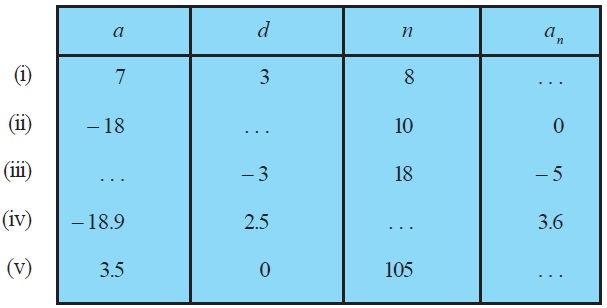Q2. Choose the correct choice in the following and justify :
(i) 30th term of the AP: 10, 7, 4, . . . , is

(A) 97   (B) 77    (C) –77    (D) – 87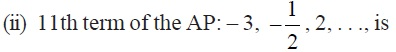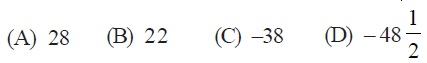Q3. In the following APs, find the missing terms in the boxes :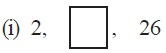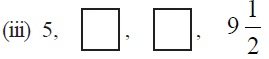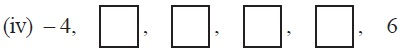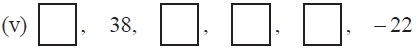Q4. Which term of the AP : 3, 8, 13, 18, . . . ,is 78?
Q5. Find the number of terms in each of the following APs :
(i) 7, 13, 19, . . . , 205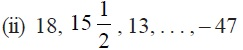Q6. Check whether – 150 is a term of the AP : 11, 8, 5, 2 . . .
Q7. Find the 31st term of an AP whose 11th term is 38 and the 16th term is 73.
Q8. An AP consists of 50 terms of which 3rd term is 12 and the last term is 106. Find the 29th term.
Q9. If the 3rd and the 9th terms of an AP are 4 and – 8 respectively, which term of this AP is zero?
Q10. The 17th term of an AP exceeds its 10th term by 7. Find the common difference.
Q11. Which term of the AP : 3, 15, 27, 39, . . . will be 132 more than its 54th term?
Q12. Two APs have the same common difference. The difference between their 100th terms is 100, what is the difference between their 1000th terms?
Q13. How many three-digit numbers are divisible by 7?
Q14. How many multiples of 4 lie between 10 and 250?
Q15. For what value of n, are the nth terms of two APs: 63, 65, 67, . . . and 3, 10, 17, . . . equal?
Q16. Determine the AP whose third term is 16 and the 7th term exceeds the 5th term by 12.

Q17. Find the 20th term from the last term of the AP : 3, 8, 13, . . ., 253.
Q18. The sum of the 4th and 8th terms of an AP is 24 and the sum of the 6th and 10th terms is 44. Find the first three terms of the AP.
Q19. Subba Rao started work in 1995 at an annual salary of Rs 5000 and received an increment of Rs 200 each year. In which year did his income reach Rs 7000?
Q20. Ramkali saved Rs 5 in the first week of a year and then increased her weekly savings by Rs 1.75. If in the nth week, her weekly savings become Rs 20.75, find n.

Page 2 of 4

Chapter Contents: